# 20 Best Online Interactive Math Games for Every Grade Level

K through 12 … it all adds up to fun!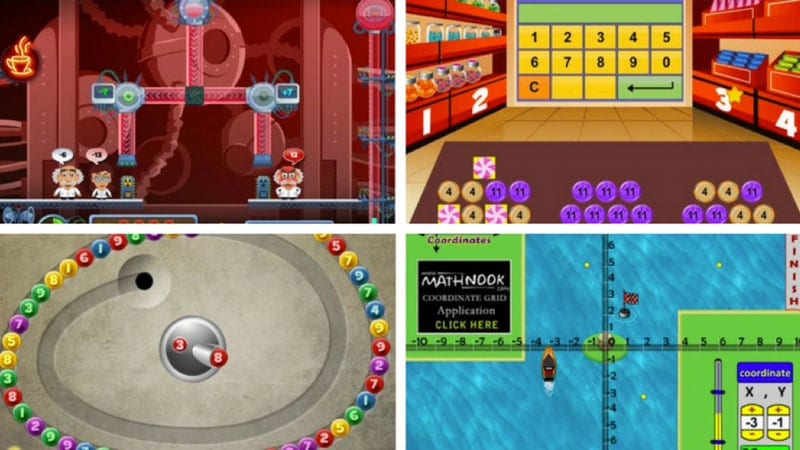### Brought to you by Epson

Want to make math games like these even more engaging and fun? Interactive projectors from EPSON can turn any surface or table into an interactive display. The included software allows you to collaborate, give feedback, and more. Learn more about our display technology options >>

More

Practice makes perfect, and math proficiency at all levels definitely takes practice. Whether students are working on basic addition and subtraction or advanced algebra and geometry concepts, these online interactive math games work as a fun way to practice important skills. The best part? They’re all available for free on the web!

While these interactive math games absolutely work with distance learning, we also love playing them on an interactive projector  because you can enlarge the display, project the game on a wall or table, and play as a class. Use your finger or the interactive pen to control the gameplay from the board. Alternatively, you can have students play the games on their individual devices in the classroom, periodically sharing their work or questions on your projector for you to give feedback.

## Best Online Interactive Math Games for Elementary School

It’s important to spark an interest in math at a young age and build confidence in skills quickly. Fun interactive math games are a great way to do it.

## Ten Frame Media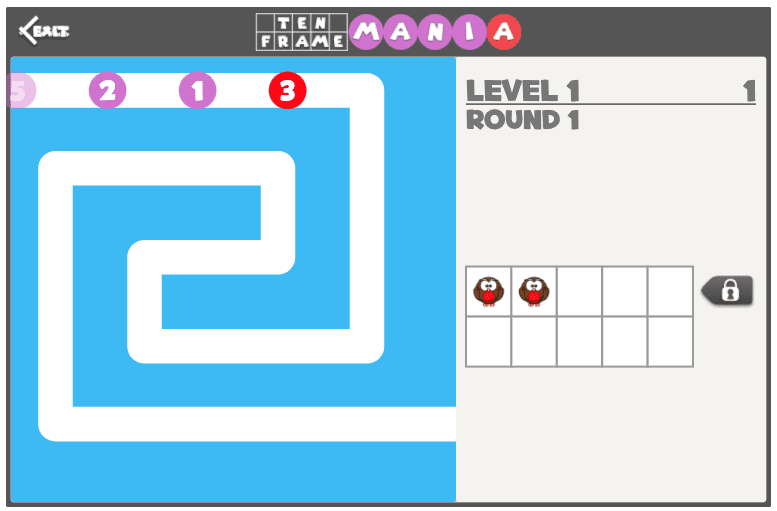• How it works: As the numbers come onto the screen, students click the boxes in the ten frame to match the number. It disappears and they start over with the next number!
• What students practice: Counting and ten frames
• Where to find it: GregTangMath

## Puzzle Pics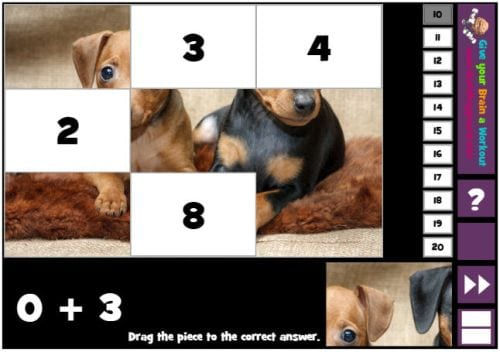• How it works: Click and drag the puzzle pieces to answer the math questions, revealing a cool picture at the end. There are multiple versions for different skills and levels, and you can change the target sums too.
• What students practice: Basic addition and subtraction
• Where to find it: Math Playground

## Bar Graphing With Eggs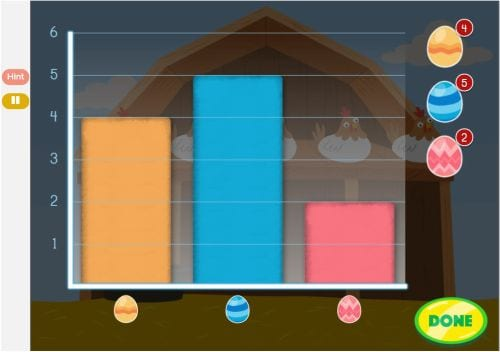• How it works: First, move the frying pan to catch the colored eggs as they fall from the chickens. Then, sort the eggs by pattern. Finally, use the eggs to create a basic bar graph.
• What students practice: Sorting, graphing
• Where to find it: Education.com

## Number Bonds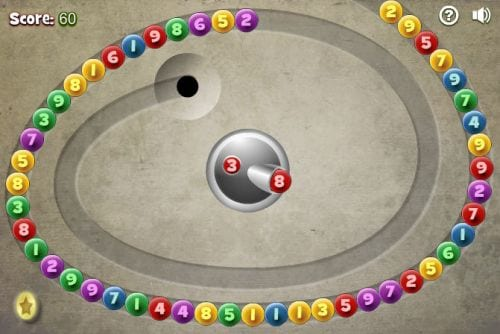• How it works: Aim and shoot the center number ball at one of the balls circling the track to make the target sum. Repeat until all the balls are gone. Different versions are available for target sums of 10, 20, 30, or 40.
• Where to find it: Math Playground

## Making Change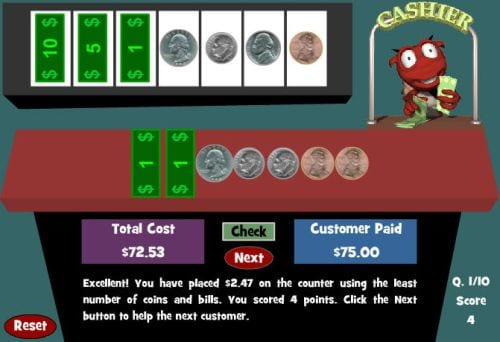• How it works: Help Harvey the cashier make change for his customers by clicking the correct coins and bills to place them on the counter. If you get the wrong answer, the computer prompts you to correct your error.
• What students practice: Subtraction, money skills
• Where to find it: Math Playground

## Fruit Splat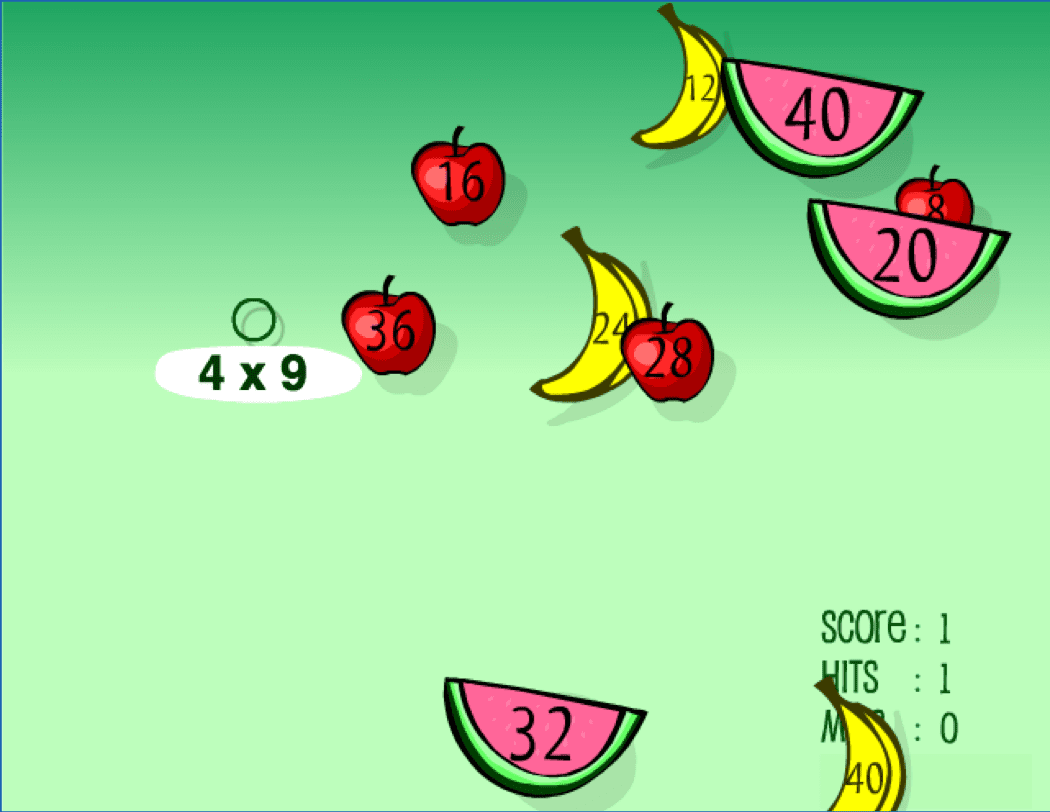• How it works: Students solve multiplication and division problems by “splatting” pieces of fruit.
• What students practice: Multiplication, division
• Where to find it: Sheppard Software

## Coin Weighing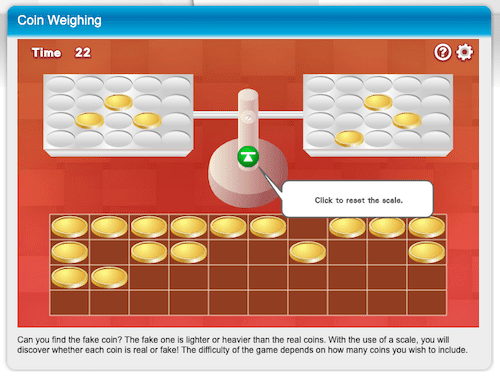• How it works: Students have four chances to weigh coins to determine which one is fake.
• What students practice: Comparison, weights
• Where to find it: Math Game TIme

## Best Online Interactive Math Games for Middle School

As math becomes more challenging, it can also become more difficult to have student engagement. Entertaining math games help students sharpen their skills without feeling like a bore.

## Puppy Chase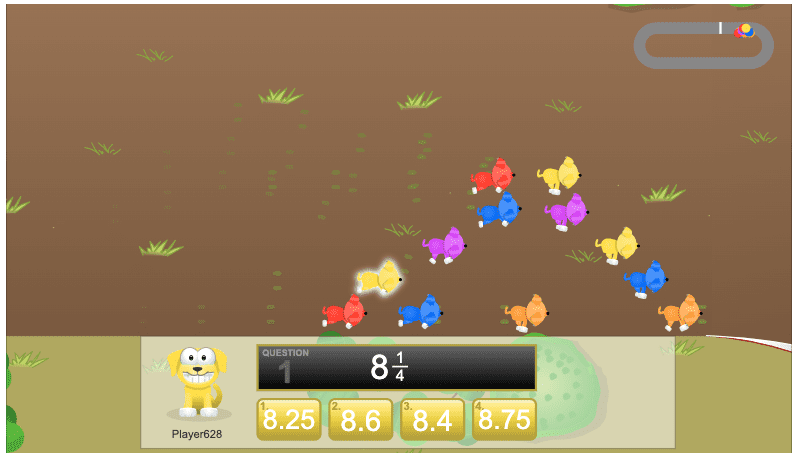• How it works: Click the equivalent decimal from the fraction given. The faster you find the answers, the more you move ahead to win the race!
• What students practice: Decimals and equivalent fractions
• Where to find it: Math Playground

## Find the Quark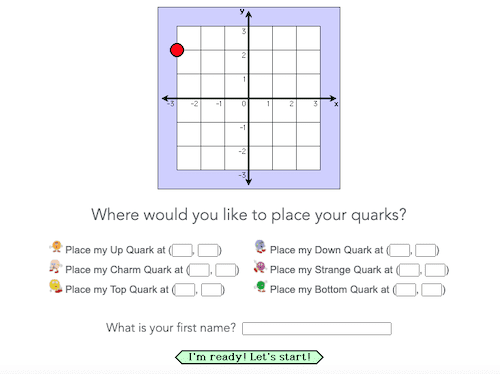• How it works: Using coordinates, this game is a bit like battleship!
• What students practice: Coordinate graphing
• Where to find it: JLab

## High Stakes Heist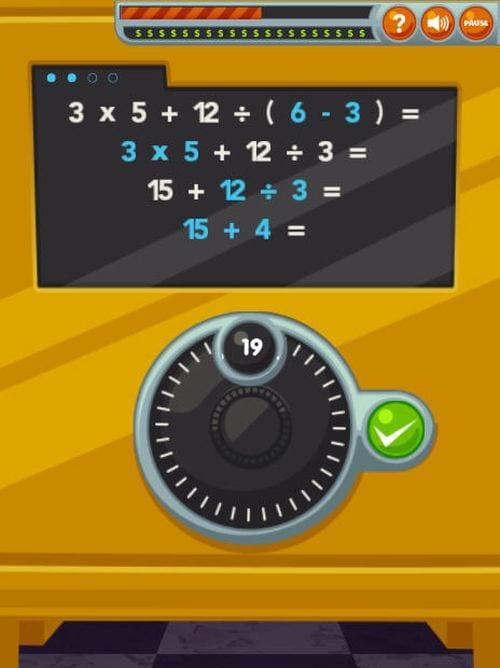• How it works: Crack the safe by solving an equation, one step at a time, using the correct order of operations. If you make a mistake, you can go back to the previous step to fix it.
• What students practice: Order of operations
• Where to find it: ABCYa

## Integers Blocks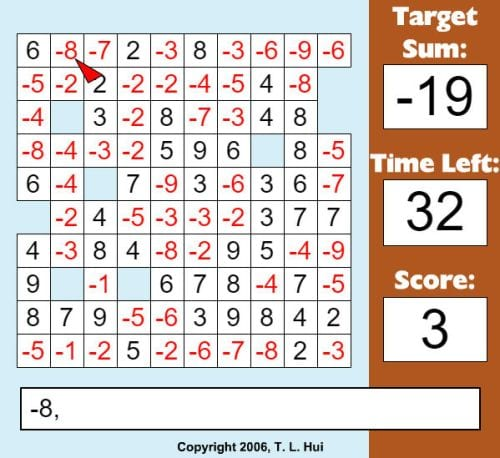• How it works: In this timed game, click the numbers that add up to or are the product of the target number, which changes each time you reach it. Blocks disappear as you click them, and you may need to select multiple numbers to reach your goal. Addition and multiplication versions are available.
• What students practice: Addition and multiplication with rational numbers
• Where to find it: XP Math

## Algebraic Reasoning Sweet Shop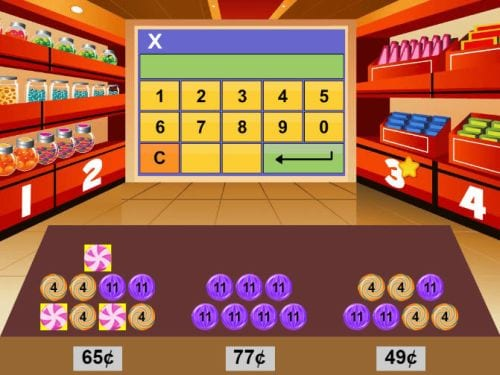• How it works: Using the stacks of various candies that add up to certain amounts, determine how much each individual candy is worth. This can be done as mental math or by writing out and solving equations.
• What students practice: Mental math, writing equations
• Where to find it: Math Playground

## Boat Coordinates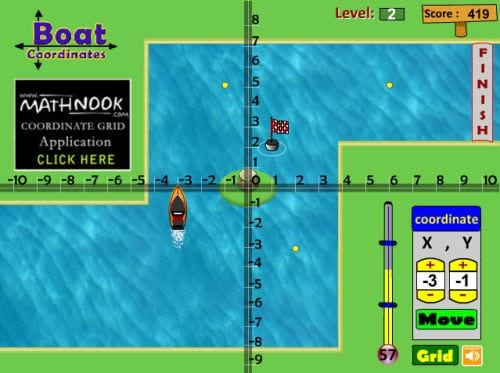• How it works: Enter the coordinates to send the boat on its way to the finish line. Stop along the way to pick up coins but be sure to avoid obstacles. You have a limited amount of time, so think fast! This game can be customized—make single-quadrant or four-quadrant boards.
• What students practice: Coordinate graphing
• Where to find it: Math Nook

## Algebra Meltdown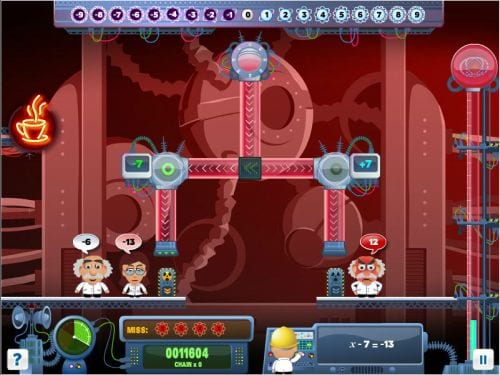• How it works: Solve linear equations and drop the correct number of balls into the reactor to give each scientist the number of atoms they need. The game is simple at first but gets more complicated as you advance. Work quickly, or the scientists will get mad and take away your lives.
• What students practice: Linear equations
• Where to find it: Mangahigh

## Best Online Interactive Math Games for High School

Higher level math doesn’t have to be all work and no play. Online math games for high school students are harder to find, but these games give students the chance to practice their skills in a unique way.

## Wrecks Factor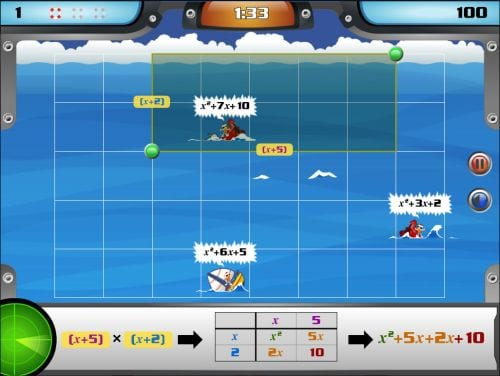• How it works: Ships sail onto the board and wreck, displaying a quadratic equation. Factor the equation, then click and drag the correct answer to the points on the grid that surround the boat. More boats continue to arrive, and if one sinks before you solve the equation, you lose a life.
• What students practice: Factoring quadratic equations
• Where to find it: Mangahigh

## Portal Transformation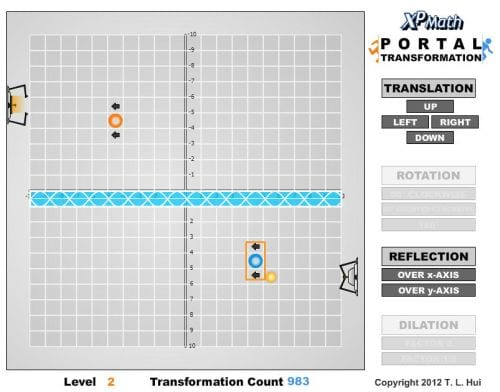• How it works: Translate and transform the portals around the coordinate plane to transport the pellet into the receptacle. Some are simple, while others involve transformations like reflection, rotation, and dilation.
• What students practice: Translation and transformation in a coordinate plane
• Where to find it: XP Math

## Transformation Golf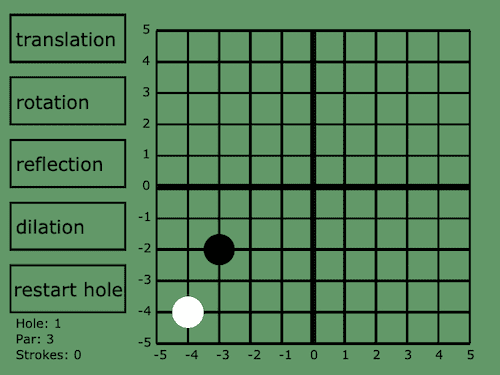• How it works: Pick a transformation and then a factor choice of that transformation to get the golf ball into the hole. Includes translation, rotation, reflection, and dilation.
• What students practice: Translation and transformation in a coordinate plane
• Where to find it: Hooda Math

## Sortify: Angles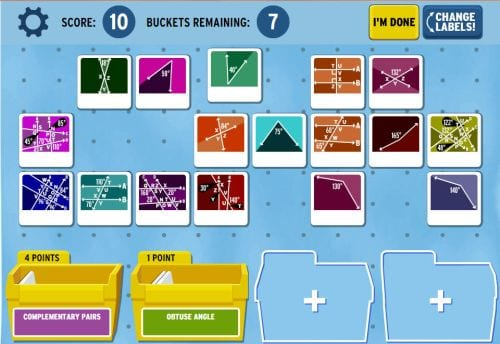• How it works: Sort the cards by dragging them into bins and placing the correct label on them. Some cards need to be linked together, like those that form complementary or supplementary angles. Submit the bins to be checked and earn points for correct answers.
• What students practice: Types of angles, basic geometry
• Where to find it: Brainpop

## Trigonometry Minigolf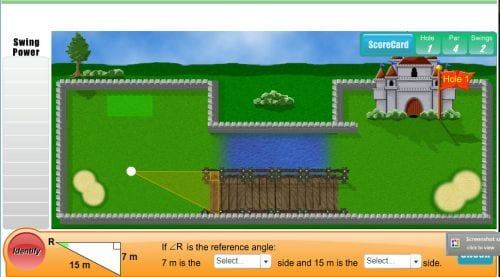• How it works: Answer the trigonometry questions to work your way through a miniature golf course. More correct answers will improve your swing power. For incorrect answers, the game explains your mistakes and allows you to try again.
• What students practice: Calculating sine, cosine, and tangent
• Where to find it: Learn Alberta

## Geogebra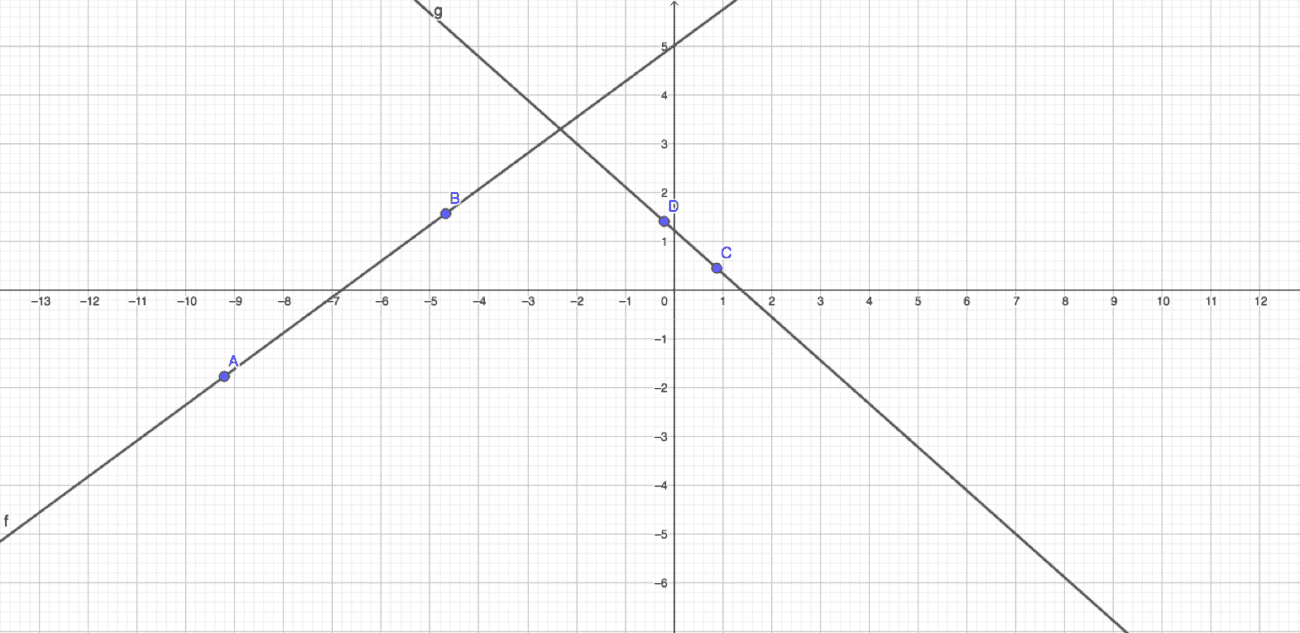• How it works: While not a game per se, we love the interactive site Geogebra for creating graphs, 3D shapes, and more. It’s a perfect way to add an interactive touch to your geometry lessons.
• What students practice: Geometry
• Where to find it: Geogebra

Take these online interactive math games to the next level by using them with your interactive projector. We love the options available from EPSON.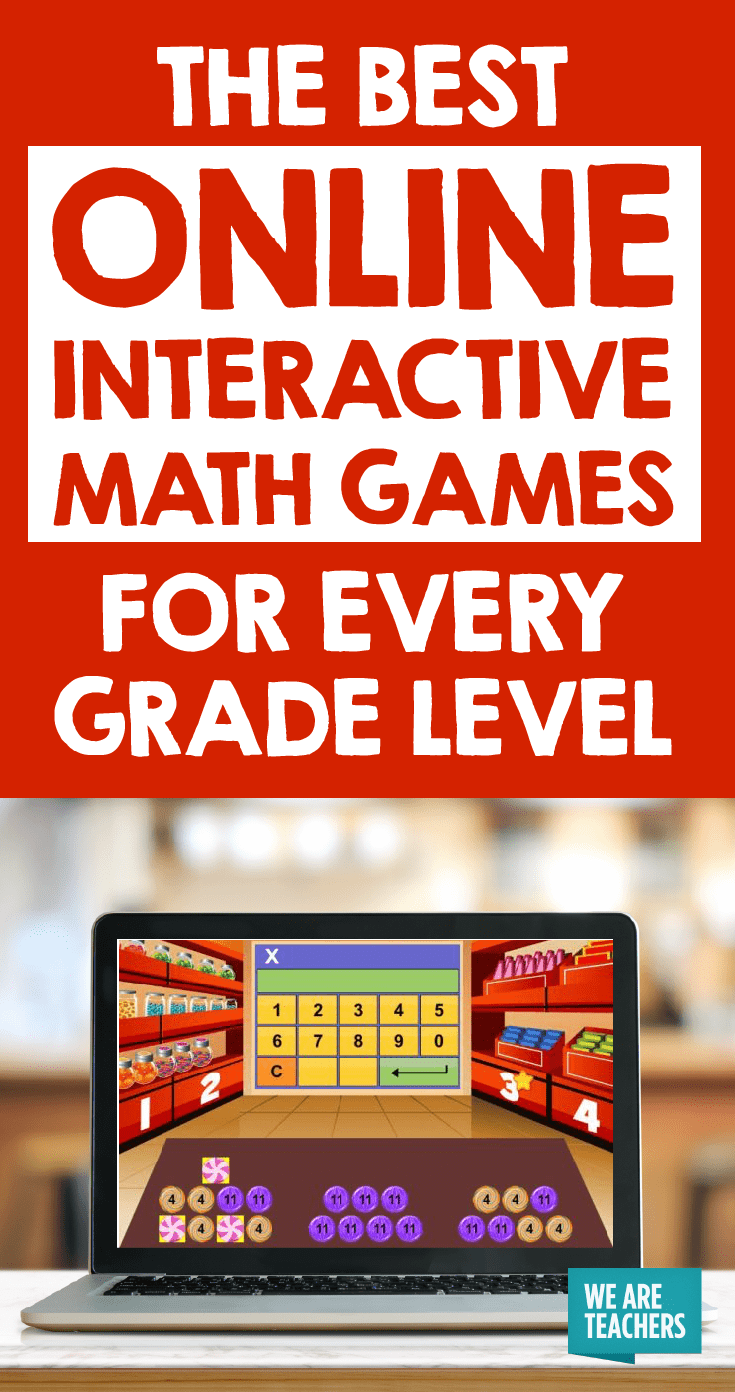### Posted by Jill Staake

Jill Staake is a writer living in Tampa, Florida. She's spent most of her life teaching in traditional classrooms and beyond, from 8th grade English to butterfly encounters, and believes learning is a life-long process.

+